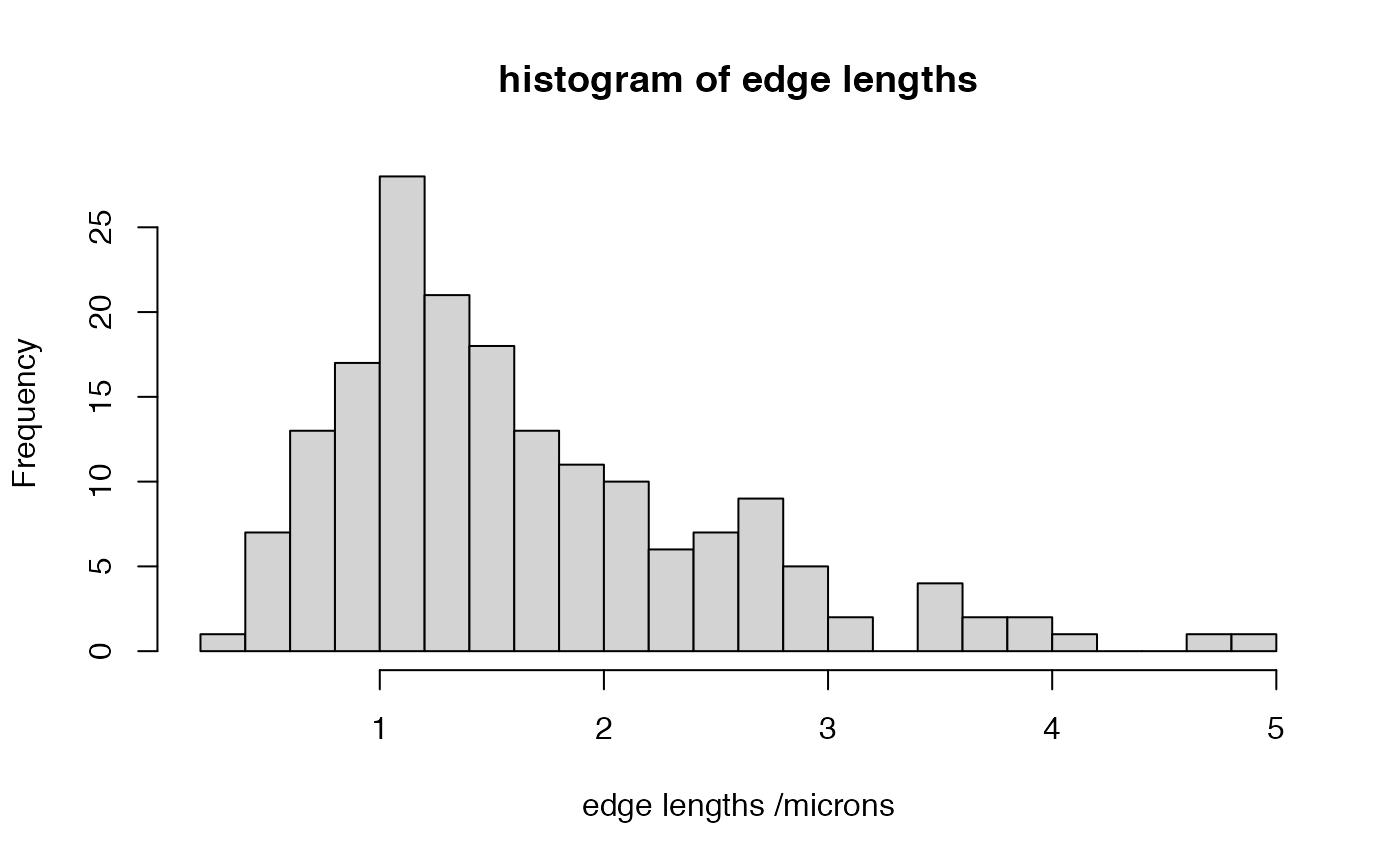Calculate length of all segments in neuron

seglengths(x, all = FALSE, flatten = TRUE, sumsegment = TRUE)

## Arguments

x

A neuron

all

Whether to calculate lengths for all segments when there are multiple subtrees (default: FALSE)

flatten

Whether to flatten the lists of lists into a single list when all=TRUE

sumsegment

Whether to return the length of each segment (when sumsegment=TRUE, the default) or a list of vectors of lengths of each individual edge in the segment.

## Value

A vector of lengths for each segment or when sumsegment=FALSE a list of vectors

## Details

A segment is an unbranched portion of neurite consisting of at least one vertex joined by edges.Only segments in x\$SegList will be calculated unless all=TRUE. Segments containing only one point will have 0 length.

as.seglist.neuron
summary(seglengths(Cell07PNs[]))